Select Page

# Accounting Equation

Accounting Equation is also called Balance Sheet Equation. It is a basic concept of agreement between left-hand and right-hand site and starting pint of double entry. Double Entry Accounting states that for every debit entry there should be pass a credit entry. Every transaction has twofold effect; this concept has a result of accounting equation or fundamental equation. At any point of time total Assets must be equal to equities. In other words we can say that left hand side which is resource side must be equal to right hand side which is of course source side.

#### Left hand side = Right hand side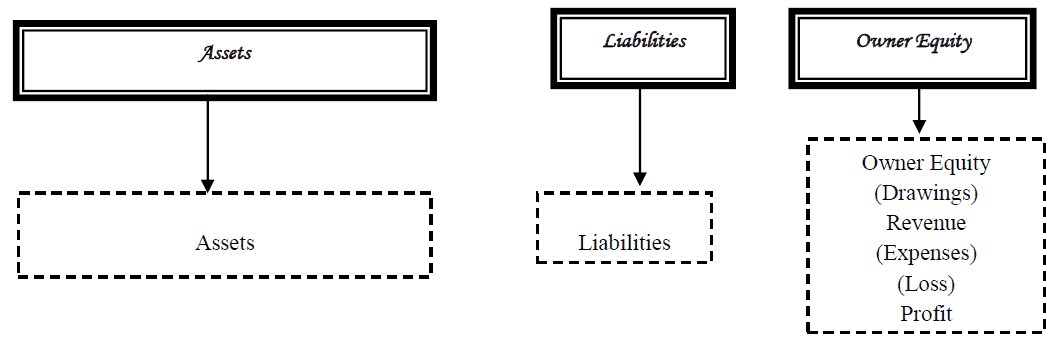## Different Presentation of Accounting Equation

There are different style of presentation mention below for balance sheet equation: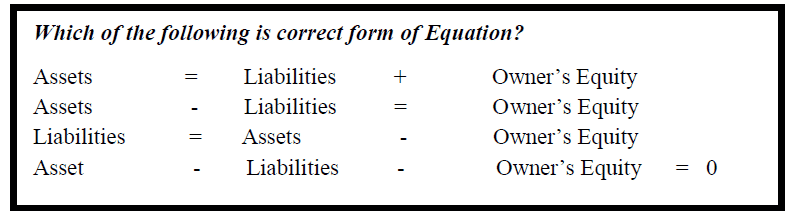>> Practice Questions with the help of Accounting Equation Format.

## Four Effects of Transactions

Accounting Transaction is broadly classified into four categories:

## Expanded Accounting Equation

The Expanded Accounting Equation of sole proprietorship and partnership is Accounting Equation (Assets = Liabilities + Owner’s Equity) – Expenses + Revenue – Drawings Account.

In case of company business the expanded accounting equation in the balance sheet equation (Assets = Liabilities + Stockholders’ Equity) with replacement of Stockholders’ Equity with Paid-in Capital – Expenses + Revenues – Treasury Stock – Dividends.

> > Practice Accounting Equation Problems and Solutions.

### Example # 1:

For each of the following transactions indicate the effects i.e. (Increase, Decrease, Conversion or No Effect)?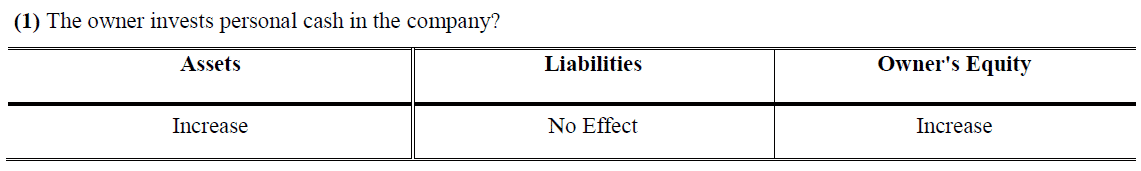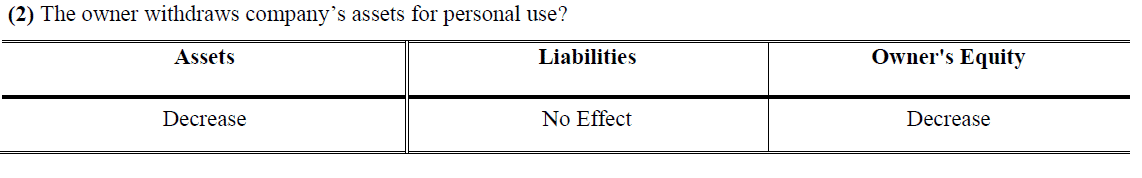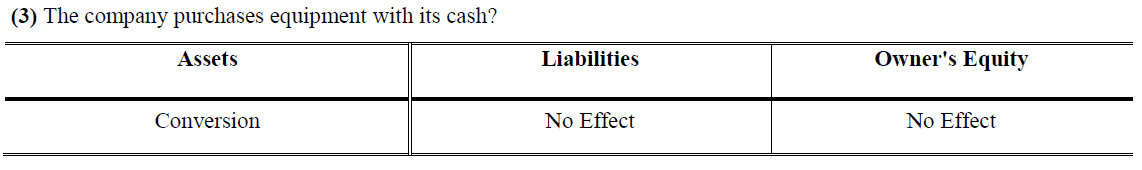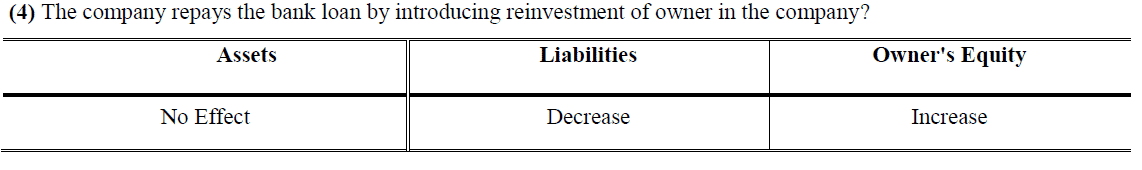### Example # 2:

From the list of accounts below, determine which assets are and which equities are.  List the assets under the Asset Column and the equities under the equities Column.  Then add each column and complete the Fundamental Accounting Equation?

 Accounts Asset Equities Cash Rs. 5,000 Rs. 5,000 Accounts Receivable 10, 600 10,600 Building 35,000 35,000 Accounts Payable 5,000 5,000 Inventory 200 200 Computer Equipment 18,000 18,000 Bank Loan 6,000 6,000 Mortgage Payable 10,000 10,000 Owner Equity 47,800 47,800 TOTAL Rs. 137,600 Rs. 68,800 Rs. 68,800

### Example # 3:

For each of the following equations, a figure is missing.  In the space provided, please supply the missing figure that will balance the equation.

 Assets Liabilities Owner’s Equity 100 20.00 80 790 720 70 2,000 400 1,600 11,218 10,000 1,218 1,010 25 985 340 12 328 8793 0 8793 2,000 1,501 499

>> Practice Accounting Equation Quiz 1 and Quiz 2.

1.